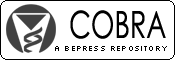## COBRA Preprint Series

#### Abstract

The propensity score analysis is one of the most widely used methods for studying the causal treatment effect in observational studies. This paper studies treatment effect estimation with the method of matching weights. This method resembles propensity score matching but offers a number of new features including efficient estimation, rigorous variance calculation, simple asymptotics, statistical tests of balance, clearly identified target population with optimal sampling property, and no need for choosing matching algorithm and caliper size. In addition, we propose the mirror histogram as a useful tool for graphically displaying balance. The method also shares some features of the inverse probability weighting methods, but the computation remains stable when the propensity scores approach 0 or 1. An augmented version of the matching weight estimator is developed that has the double robust property, i.e., the estimator is consistent if either the outcome model or the propensity score model is correct. In the numerical studies, the proposed methods demonstrated better performance than many widely used propensity score analysis methods such as stratification by quintiles, matching with propensity scores, and inverse probability weighting.

#### Disciplines

Statistical Methodology | Statistical Theory

COinS